【1】NN复杂度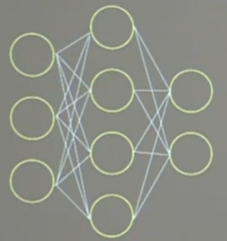【2】指数衰减学习率

epoch = 40
LR_BASE = 0.2  # 最初学习率
LR_DECAY = 0.99  # 学习率衰减率
LR_STEP = 1  # 喂入多少轮BATCH_SIZE后，更新一次学习率
for epoch in range(epoch):  # for epoch 定义顶层循环，表示对数据集循环epoch次，此例数据集数据仅有1个w,初始化时候constant赋值为5，循环100次迭代。
lr = LR_BASE * LR_DECAY ** (epoch / LR_STEP)

【3】激活函数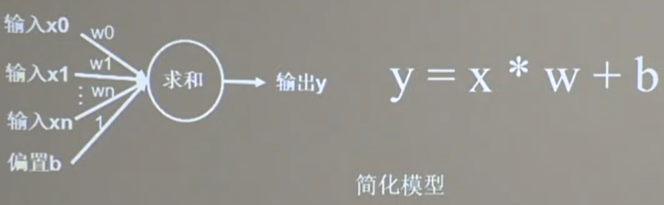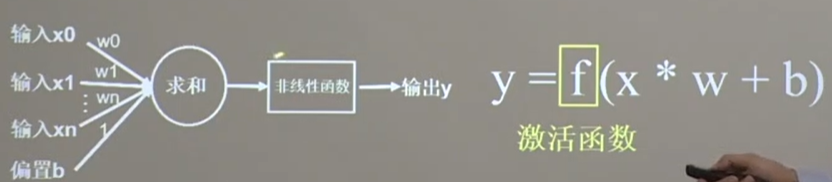MP模型比简化模型多了一个激活函数，它的加入加强了模型的表达力，使得深层网络不再是输入x的线性组合，而且随着层数增加提升表达力。

优秀激活函数所具有的特点

1、激活函数输出为有限值时，权重对于特征的影响会更显著，基于梯度的优化方法更稳定
2、激活函数输出为无限值时，参数的初始值对模型的影响特别大，要使用更小的学习率

常见的激活函数

1、sigmoid函数：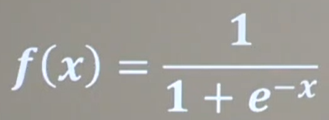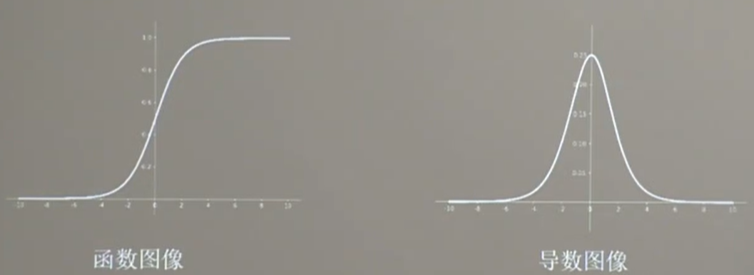1、易造成梯度消失（输入的值较大时，梯度就约等于0了）
2、输出非0均值，收敛慢（我们希望输入每层网络的特征是以0为均值的小数）
3、幂运算复杂，训练时间长

2、Tanh函数：

1、易造成梯度消失
2、输出0均值
3、幂运算复杂，训练时间长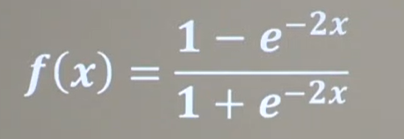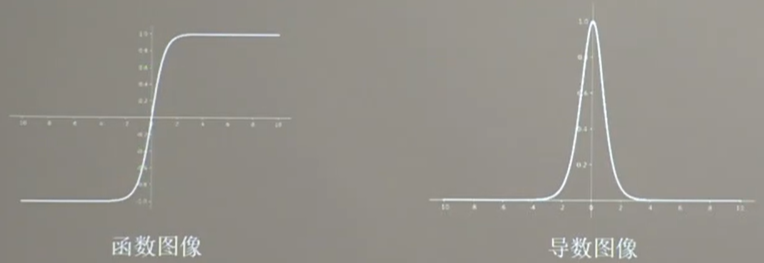3、Relu函数：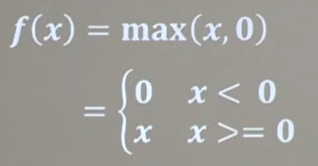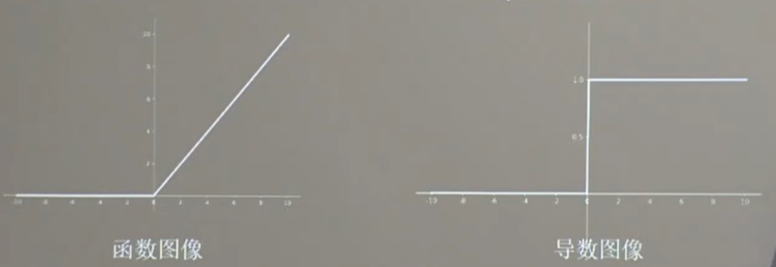4、Leaky Relu函数：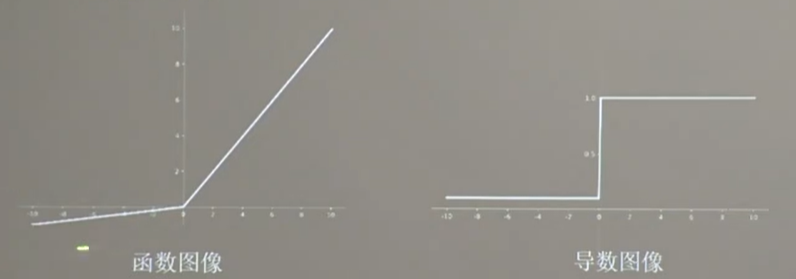对于初学者的建议

1、首选relu激活函数
2、学习率设置较小值
3、输入特征标准化，让输入特征满足以0为均值，1为标准差的正态分布
4、初始参数中心化，让随机生成的参数满足0位均值，sqrt(2/当前层输入特征个数)为标准差的正态分布

【4】损失函数

NN优化目标：loss最小

1、mse(均方差)
mse调用方式：

loss_mse =tf.reduce_mean(tf.square(y_-y))

import tensorflow as tf
import numpy as np

SEED = 23455

rdm = np.random.RandomState(seed=SEED)  # 生成[0,1)之间的随机数
x = rdm.rand(32, 2)
y_ = [[x1 + x2 + (rdm.rand() / 10.0 - 0.05)] for (x1, x2) in x]  # 生成噪声[0,1)/10=[0,0.1); [0,0.1)-0.05=[-0.05,0.05)
x = tf.cast(x, dtype=tf.float32)

w1 = tf.Variable(tf.random.normal([2, 1], stddev=1, seed=1))

epoch = 15000
lr = 0.002

for epoch in range(epoch):
y = tf.matmul(x, w1)
loss_mse = tf.reduce_mean(tf.square(y_ - y))

if epoch % 500 == 0:
print("After %d training steps,w1 is " % (epoch))
print(w1.numpy(), "\n")
print("Final w1 is: ", w1.numpy())

2、自定义损失函数loss_zdy=tf,reduce_sum(tf.where(tf.greater(y,y_),COST(y-y_),PROFIT(y_-y)))

import tensorflow as tf
import numpy as np

SEED = 23455
COST = 1
PROFIT = 99

rdm = np.random.RandomState(SEED)
x = rdm.rand(32, 2)
y_ = [[x1 + x2 + (rdm.rand() / 10.0 - 0.05)] for (x1, x2) in x]  # 生成噪声[0,1)/10=[0,0.1); [0,0.1)-0.05=[-0.05,0.05)
x = tf.cast(x, dtype=tf.float32)

w1 = tf.Variable(tf.random.normal([2, 1], stddev=1, seed=1))

epoch = 10000
lr = 0.002

for epoch in range(epoch):
y = tf.matmul(x, w1)
loss = tf.reduce_sum(tf.where(tf.greater(y, y_), (y - y_) * COST, (y_ - y) * PROFIT))

if epoch % 500 == 0:
print("After %d training steps,w1 is " % (epoch))
print(w1.numpy(), "\n")
print("Final w1 is: ", w1.numpy())

# 自定义损失函数
# 酸奶成本1元， 酸奶利润99元
# 成本很低，利润很高，人们希望多预测些，生成模型系数大于1，往多了预测

3、ce(交叉熵)

import tensorflow as tf

loss_ce1 = tf.losses.categorical_crossentropy([1, 0], [0.6, 0.4])
loss_ce2 = tf.losses.categorical_crossentropy([1, 0], [0.8, 0.2])
print("loss_ce1:", loss_ce1)
print("loss_ce2:", loss_ce2)

# 交叉熵损失函数

tf.nn.softmax_cross_entropy_with_logits(y_, y)

# softmax与交叉熵损失函数的结合
import tensorflow as tf
import numpy as np

y_ = np.array([[1, 0, 0], [0, 1, 0], [0, 0, 1], [1, 0, 0], [0, 1, 0]])
y = np.array([[12, 3, 2], [3, 10, 1], [1, 2, 5], [4, 6.5, 1.2], [3, 6, 1]])
y_pro = tf.nn.softmax(y)
loss_ce1 = tf.losses.categorical_crossentropy(y_,y_pro)
loss_ce2 = tf.nn.softmax_cross_entropy_with_logits(y_, y)

print('分步计算的结果:\n', loss_ce1)
print('结合计算的结果:\n', loss_ce2)

【5】缓解过拟合——正则化1、更好地拟合训练数据
2、保持参数尽量地小
λ过大同样也会造成欠拟合的现象。

1、增加输入特征项
2、增加网络参数
3、减少正则化参数

1、数据清洗
2、增大训练集
3、采用正则化
4、增大正则化参数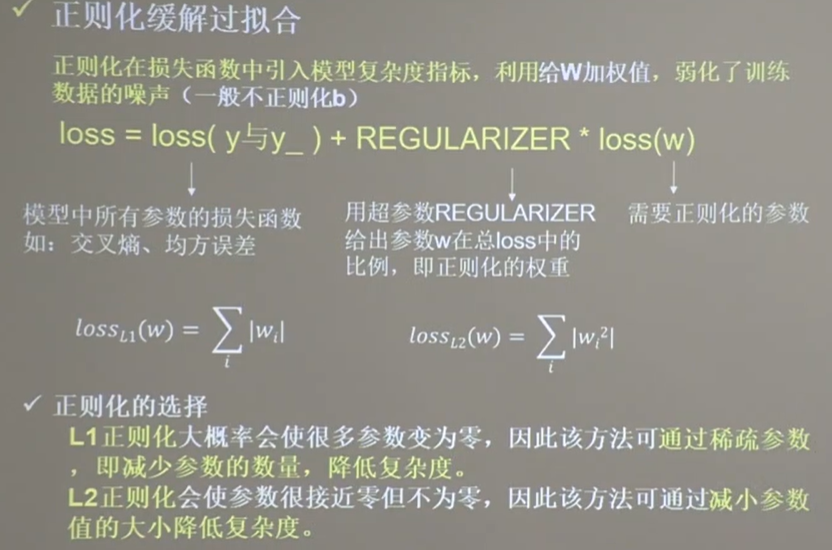1、先用神经网络拟合出数据x1,x2,y_c的函数关系
2、生成网格覆盖这些点
3、将网格中坐标送入训练好的神经网络
4、网络为每个坐标输出一个预测值
5、将神经网络输出为0.5的预测值的线标出颜色，这线就是区分线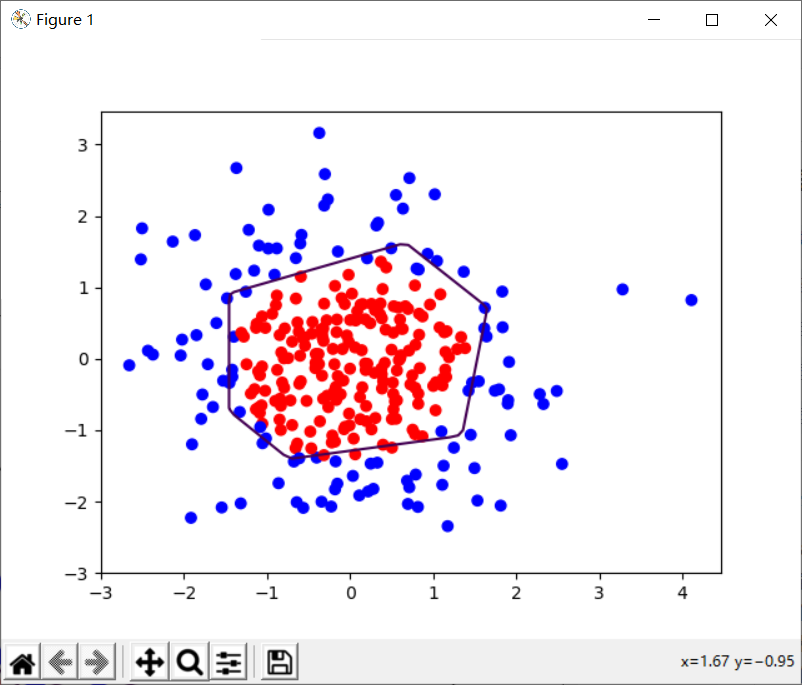1层隐藏层，4个神经元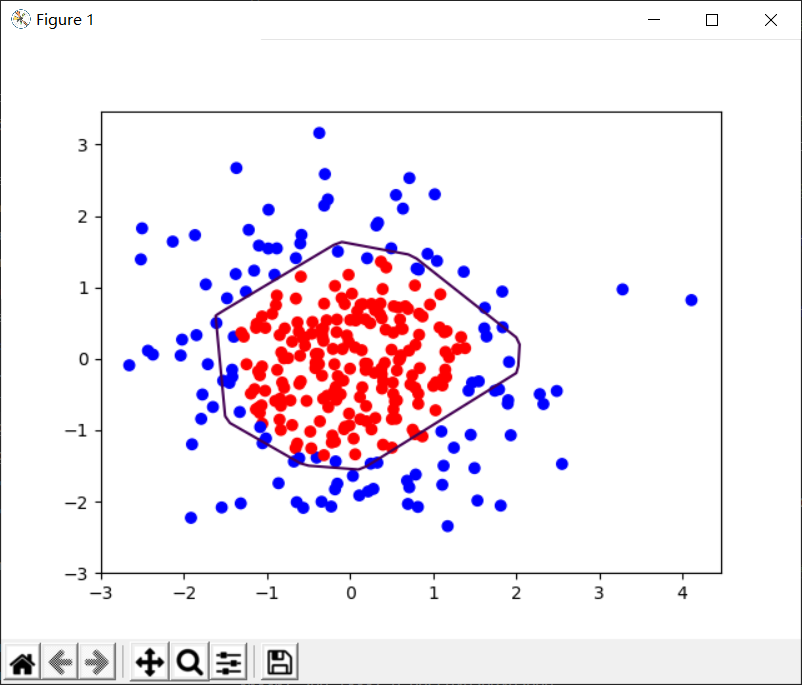1层隐藏层，4个神经元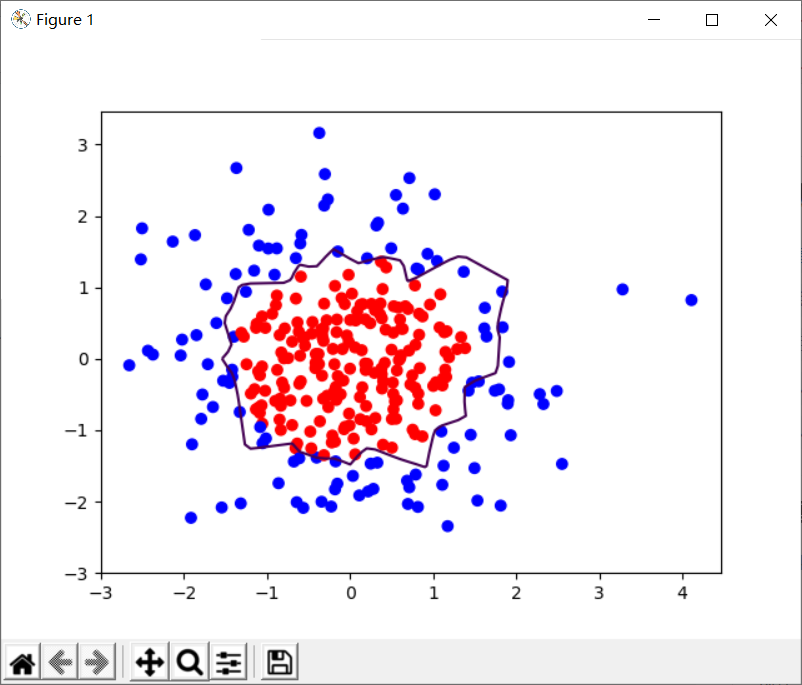1层隐藏层，22个神经元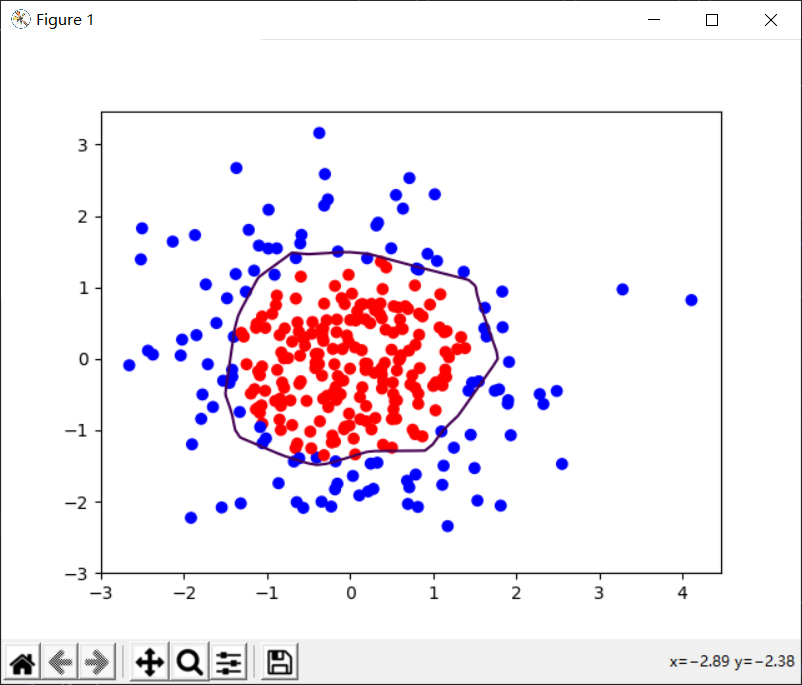1层隐藏层，22个神经元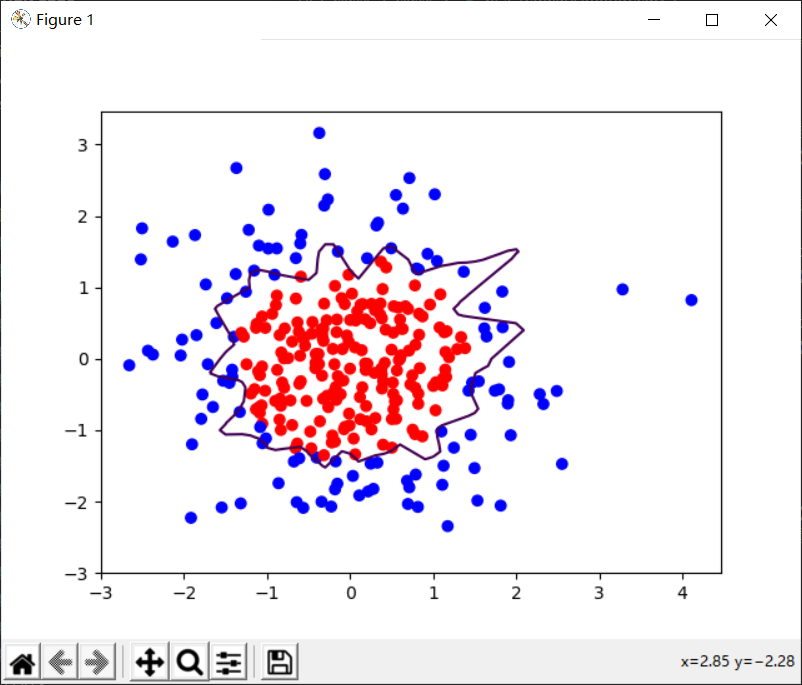1层隐藏层，40个神经元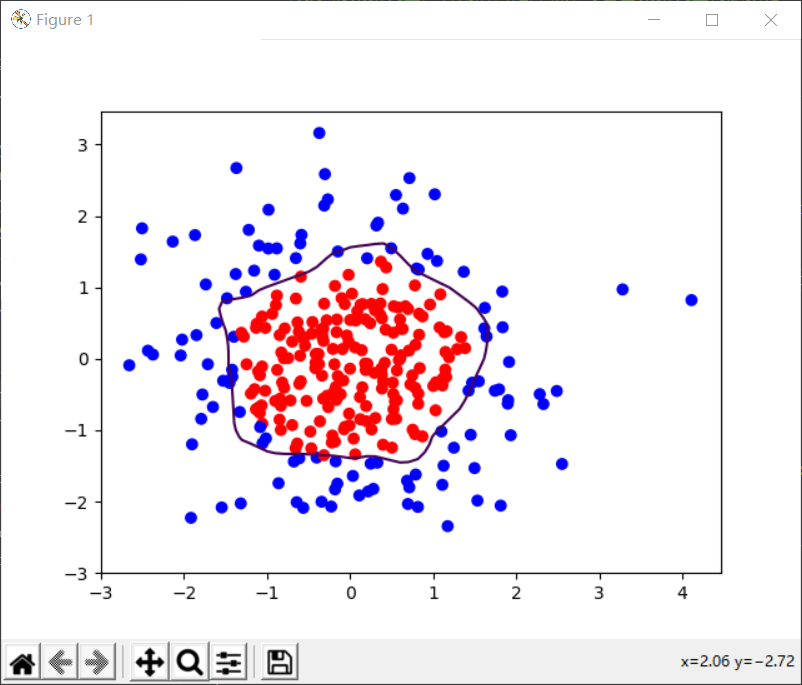1层隐藏层，40个神经元

【6】参数优化器

【基础算法】神经网络参数优化器https://zhuanlan.zhihu.com/p/97873519
MOOC神经网络参数优化器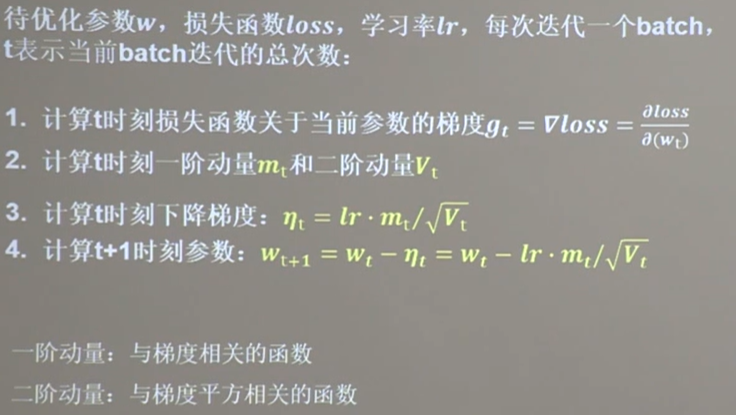【1】SGD

code：

# 实现梯度更新 w1 = w1 - lr * w1_grad    b = b - lr * b_grad

【2】SGDM(SGD基础上增加了一阶动量)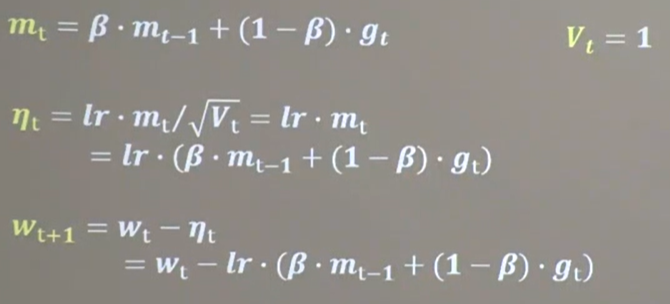mt表示各时刻梯度方向的指数滑动平均值，表征了过去一段时间的平均值。β是接近1的超参数，一般等于0.9
code:

m_w, m_b = 0, 0
beta = 0.9

# sgd-momentun
m_w = beta * m_w + (1 - beta) * grads
m_b = beta * m_b + (1 - beta) * grads
w1.assign_sub(lr * m_w)
b1.assign_sub(lr * m_b)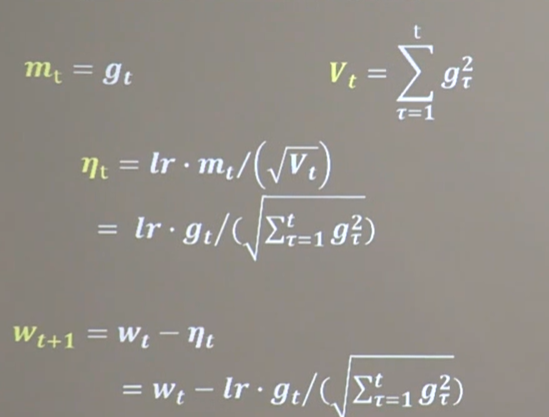code:

v_w, v_b = 0, 0

【4】RMSProp(SGD基础上增加了二阶动量)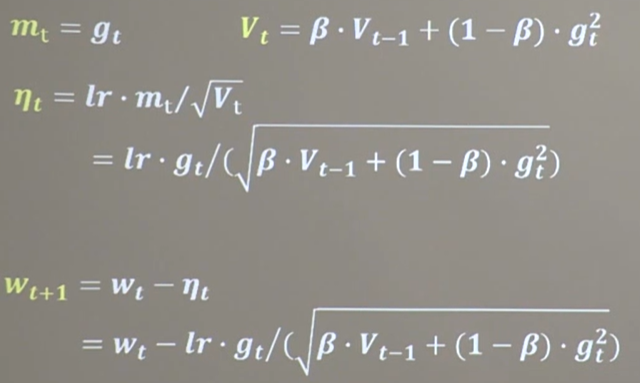v_w, v_b = 0, 0
beta = 0.9
# rmsprop
v_w = beta * v_w + (1 - beta) * tf.square(grads)
v_b = beta * v_b + (1 - beta) * tf.square(grads)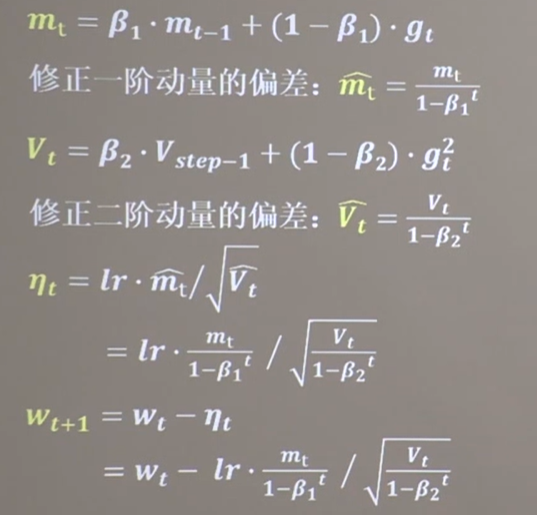code:

m_w, m_b = 0, 0
v_w, v_b = 0, 0
beta1, beta2 = 0.9, 0.999
delta_w, delta_b = 0, 0
global_step = 0
m_w = beta1 * m_w + (1 - beta1) * grads
m_b = beta1 * m_b + (1 - beta1) * grads
v_w = beta2 * v_w + (1 - beta2) * tf.square(grads)
v_b = beta2 * v_b + (1 - beta2) * tf.square(grads)

m_w_correction = m_w / (1 - tf.pow(beta1, int(global_step)))
m_b_correction = m_b / (1 - tf.pow(beta1, int(global_step)))
v_w_correction = v_w / (1 - tf.pow(beta2, int(global_step)))
v_b_correction = v_b / (1 - tf.pow(beta2, int(global_step)))

w1.assign_sub(lr * m_w_correction / tf.sqrt(v_w_correction))
b1.assign_sub(lr * m_b_correction / tf.sqrt(v_b_correction))

优化器对比总结

优化器对比

（lr=0.1    epoch=500    batch=32）